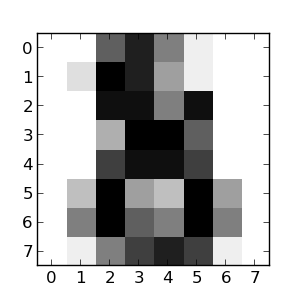This is a tutorial on classifying handwritten digits with KNN algorithm using Clatern. Clatern is a machine learning library for Clojure, in the works.

### Dataset

This tutorial uses a stripped down version of handwritten digits dataset available here. The stripped down version(taken from the sklearn library) is available here.

The dataset consists of 1797 samples of 8x8 pixels and the target labels. The first 64 columns are the 8x8 pixels and the 65th column is the label target. Let’s have a look at a sample,Splitting the data into training and test sets,

Splitting the training and test set into features and labels,

### Training

Here, we use the KNN model for classifying the digits. The syntax for KNN is,

where,
X is input data,
y is target data,
v is new input to be classified, and
k is the number of neighbours(optional, default = 3)

Let’s define a function to perform kNN on our dataset.

Now, h can be used to classify the training set.

### Testing

Let’s test the KNN model. Classifying the data in the testing set,

Now let’s check the accuracy of the model.

The model has a 99.74% accuracy on the test set! The accuracy of the model could vary highly depending on the shuffling of the dataset.

### Conclusion

The KNN model has a really good accuracy for the digit classification dataset used here. The problem with KNN is it’s inefficiency. It requires computation involving all samples in the dataset to classify a new sample. The MNIST dataset is a large dataset of handwritten digits - 50,000 training set and 10,000 test set samples. A more complex model such as SVM or MLP(Multi Layer Perceptron) may be used for better efficiency and classification accuracy for such datasets. That’s it! more work on Clatern to follow soon. So, keep an eye out :-)